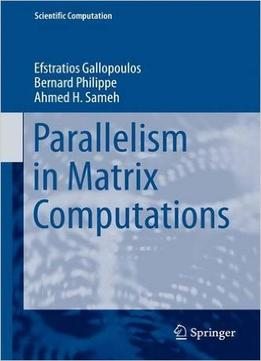# Parallelism In Matrix Computations by Bernard Philippe / 2015 / English / PDF

This book is primarily intended as a research monograph that could also be used in graduate courses for the design of parallel algorithms in matrix computations. It assumes general but not extensive knowledge of numerical linear algebra, parallel architectures, and parallel programming paradigms. The book consists of four parts: (I) Basics; (II) Dense and Special Matrix Computations; (III) Sparse Matrix Computations; and (IV) Matrix functions and characteristics. Part I deals with parallel programming paradigms and fundamental kernels, including reordering schemes for sparse matrices. Part II is devoted to dense matrix computations such as parallel algorithms for solving linear systems, linear least squares, the symmetric algebraic eigenvalue problem, and the singular-value decomposition. It also deals with the development of parallel algorithms for special linear systems such as banded ,Vandermonde ,Toeplitz ,and block Toeplitz systems. Part III addresses sparse matrix computations: (a) the development of parallel iterative linear system solvers with emphasis on scalable preconditioners, (b) parallel schemes for obtaining a few of the extreme eigenpairs or those contained in a given interval in the spectrum of a standard or generalized symmetric eigenvalue problem, and © parallel methods for computing a few of the extreme singular triplets. Part IV focuses on the development of parallel algorithms for matrix functions and special characteristics such as the matrix pseudospectrum and the determinant. The book also reviews the theoretical and practical background necessary when designing these algorithms and includes an extensive bibliography that will be useful to researchers and students alike. The book brings together many existing algorithms for the fundamental matrix computations that have a proven track record of efficient implementation in terms of data locality and data transfer on state-of-the-art systems, as well as several algorithms that are presented for the first time, focusing on the opportunities for parallelism and algorithm robustness.

views: 593